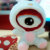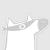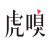0+0+0+Redmi #红米电视#采用的系统与小米电视一样，对此，官微在评论回应道：“我们就是100%用小米电视 的PatchWall（拼图墙）人工智能系统，真的很好用哦”0+0+0+0+0+0+0+0+0+0+0+0+0+0+0+0+0+0+0+0+0+0+0+0+0+1+0+0+0+0+0+0+0+0+0+0+0+0+0+【李笛：#人工智能必须无所不能吗#？】

#虎嗅FM创新节#上，微软（亚洲）互联网工程院副院长、小冰团队总负责人李笛谈到人工智能时表示：大家都知道科学家们的IQ比较高，EQ比较低，所以大家通常会想要去尝试用一下两种方式来搭建我们的人工智能系统。第一种：让它做到无所不能，第二种：希望它能够做...全文0+0+0+
Top